Worksheet Question: Basic Geometrical Ideas

# Worksheet Question: Basic Geometrical Ideas - Notes | Study Mathematics (Maths) Class 6 - Class 6

 1 Crore+ students have signed up on EduRev. Have you?

Ques 1: What do the following things in our surrounding represent: (use the words given in the box).

 A point A ray A line segment A plane Parallel lines Intersecting lines

Tip of pencil A point (example)
(a) Edge of a ruler __________
(b) Corner of a book __________
(c) Light coming out of torch __________
(d) Flat surface of a table __________
(e) Opposite edges of a ruler __________
(f) Adjacent edges of a ruler __________

Ans: Basic Geometrical Ideas
(a) Line segment
(b) Point
(c) Ray
(d) Plane
(e) Parallel lines
(f) Intersecting lines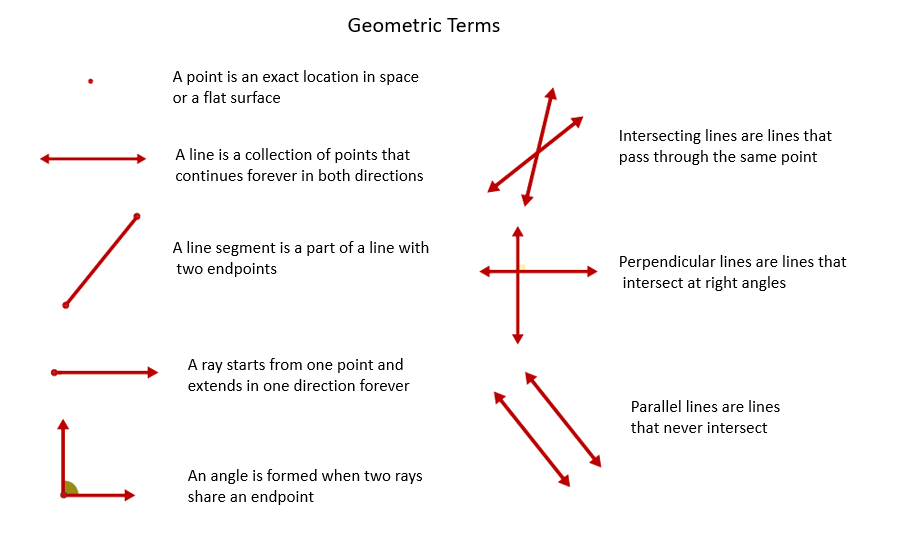Types of lines

Ques 2: In relation to the adjoining figures, write the names of: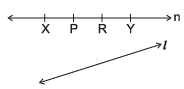(a) 6 line segments
(b) 6 rays
(c) Two intersecting lines
(d) Two parallel lines
(e) How many points have been marked and named on the line 'n'?
(f) How many points does a line have?

Ans: (a) Line segments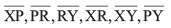(b) Rays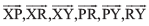(c) Line 'n' and line 'l'
(d) line 'm' and line 'n'
(e) 4
(f) Infinitely many

Ques 3: Fill in the blanks, according to the given figure:
(a) Shaded region is representing __________.
(b) __________  is a diameter of the circle.
(c) __________  is a chord of the circle.
(d) __________  is a radius of the circle.
(e) Line segment PO = line segment __________  (in length)
(f) PR is dividing the circle into two equal halves, and each half is called __________.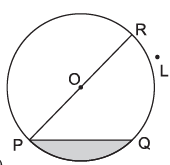Ans: (a) Segment of a circle
(b) PR
(c) PQ
(d) OP or OR
(e) RO
(f) Semi-circle
(g) Point L

Ques 4: State whether the following statements are true or false:
(a) Sector of a circle is the region in the interior of a circle enclosed by an arc on one side and a pair of radii on the other two sides.
(b) Circle is a polygon.
(c) A line is also a curve.
(d) Interior of an angle is a restricted area.
(e) Line is a part of a ray.
(f) Two lines in a plane can intersect each other at two points.
(g) Line segment is a definite part of a line.
(h) Through a given piont, only one line can be drawn.
Ans:
(a) True
(b) False
(c) True
(d) False
(e) False
(f) False
(g) True
(h) False

Ques 5: For quadrilateral ABCD, write
(a) Other name of this quadrilateral.
(b) One pair of opposite sides.
(c) One pair of adjacent sides.
(d) One pair of adjacent angles.
(e) One pair of opposite angles.
(f) One pair of adjacent vertices.
(g) Name of diagonals.
(h) Name of the triangle whose one angle is ∠DAC.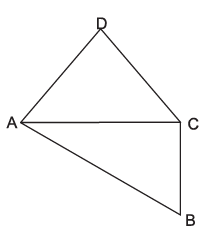Ans: (a) BCDA or CDAB (any 1 name)
(b) AD and BC; AB and CD (any 1 pair)
(c) AB and BC; BC and CD; CD and AD; AD and AB. (any 1 pair)
(d) ∠DAB and ∠ABC; ∠ABC and ∠BCD; ∠BCD and ∠CDA; ∠CDA and ∠DAB. (any 1 pair)
(e) ∠ABC and ∠ADC; ∠DAB and ∠DCB (any 1 pair)
(f) A and B; B and C; C and D; D and A. (any 1 pair)
(g) AC and BD
(h) ∆ADC or ∆DAC or ∆DCA (any 1)

Ques 6: Match the following:

 (a) Open curve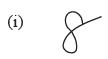(b) Closed curve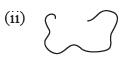(c) Polygon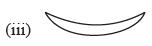(d) Not a simple curve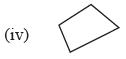Ans: (a) ↔ (ii),
(b) ↔ (iii),
(c) ↔ (iv),
(d) ↔ (i)

The document Worksheet Question: Basic Geometrical Ideas - Notes | Study Mathematics (Maths) Class 6 - Class 6 is a part of the Class 6 Course Mathematics (Maths) Class 6.
All you need of Class 6 at this link: Class 6

## Mathematics (Maths) Class 6

168 videos|282 docs|43 tests
 Use Code STAYHOME200 and get INR 200 additional OFF

## Mathematics (Maths) Class 6

168 videos|282 docs|43 tests

Track your progress, build streaks, highlight & save important lessons and more!

,

,

,

,

,

,

,

,

,

,

,

,

,

,

,

,

,

,

,

,

,

;